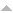## Publications

Export 3 results:
Sort by: Author [ Title] Type Year
O
Cipriano, Fernanda. "On the asymptotic behaviour and stochastic stabilization of second grade fluids." Stochastics (In Press): 1-21. AbstractWebsite

ABSTRACTIn this article, we study stability properties for two-dimensional non-Newtonian fluids. More precisely, we consider stochastic perturbations of the second grade fluid equations, with Navier slip boundary condition, and analyse the asymptotic behaviour of the solutions as t→+∞. We prove that the strong solutions (in the probability sense) of the stochastic evolutionary equation converge exponentially to the stationary solution in the mean square and almost surely. In addition, we study the stabilization of the deterministic model by introducing a suitable stochastic noise.

Cipriano, Fernanda, and Diogo Pereira. "On the existence of optimal and ϵ−optimal feedback controls for stochastic second grade fluids." Journal of Mathematical Analysis and Applications. 475 (2019): 1956-1977. AbstractWebsite

This article deals with a feedback optimal control problem for the stochastic second grade fluids. More precisely, we establish the existence of an optimal feedback control for the two-dimensional stochastic second grade fluids, with Navier-slip boundary conditions. In addition, using the Galerkin approximations, we show that the optimal cost can be approximated by a sequence of finite dimensional optimal costs, showing the existence of the so-called ϵ−optimal feedback control.

Chemetov, Nikolai, and Fernanda Cipriano. "Optimal control for two-dimensional stochastic second grade fluids." STOCHASTIC PROCESSES AND THEIR APPLICATIONS. 128 (2018): 2710-2749. Abstract

{This article deals with a stochastic control problem for certain fluids of non-Newtonian type. More precisely, the state equation is given by the two-dimensional stochastic second grade fluids perturbed by a multiplicative white noise. The control acts through an external stochastic force and we search for a control that minimizes a cost functional. We show that the Gateaux derivative of the control to state map is a stochastic process being the unique solution of the stochastic linearized state equation. The well-posedness of the corresponding stochastic backward adjoint equation is also established, allowing to derive the first order optimality condition. (C) 2017 Elsevier B.V. All rights reserved.}